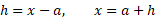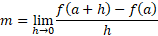It looks like you're using Internet Explorer 11 or older. This website works best with modern browsers such as the latest versions of Chrome, Firefox, Safari, and Edge. If you continue with this browser, you may see unexpected results.

# Math

Some of the content of this guide was modeled after a guide originally created by Openstax and has been adapted for the GPRC Learning Commons in September 2021. The graphs are generated using Desmos. This work is licensed under a Creative Commons BY 4.0 International License.## Tangent Lines

##### Calculus is the study of functions and how they relate to the world around us.  The development of calculus started with the tangent of a curve and finding the area underneath a curve.  For this guide, we will be starting with a secant line and working towards solving for a tangent line.

The secant line of a curve is:

"an intersecting line, especially one intersecting a curve at two or more points."

From <https://www.dictionary.com/browse/secant>

The tangent line of a curve is:

"a line or a plane that touches a curve or a surface at a point so that it is closer to the curve in the vicinity of the point than any other line or plane drawn through the point."

From <https://www.dictionary.com/browse/tangent>The above graph shows the tangent line for point (0.5,0.125) for f(x)=x³.  We will go through the visuals below to show how we use varying points and the slope of the secants to approach the tangent at this point.

The first step is to define a point near (a,f(a)) and calculate the slope of the secant line through these two points.  We use the line equation to find this slope by taking the ratio of the difference in y-values by the difference in x-values.  This is also known as "rise over run" as it indicates how far up or down the line "rises" on the y-axis as the x-value "runs" along the x-axis.  Also, for the purpose of this example, we will be leaving values in terms of points (a,f(a)) and (c,f(c)), to act as the definition of our limit.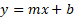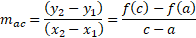After finding the slope of our secant , we can use the line equation to substitute in for known values, ac, a, and f(a), and solve for the secant line equation.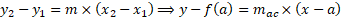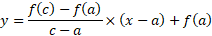As we view points closer and closer to a, the secant line trends closer and closer to the expected tangent line:If we proceed beyond our point, the secant line trends away from the expected tangent line once again:If we let the point (x,f(x)) approach the fixed point (a,f(a)), and the slope ac approaches a value m, then we define the tangent of point (a,f(a)) to be limit of the slopes of the secant lines through point (a,f(a)) with a slope of m.  This limit can be represented in the following form: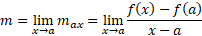For some purposes, it is convenient to rewrite the formula as such: